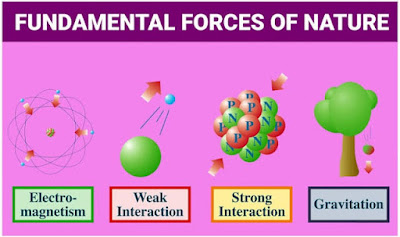## Posts

Showing posts from August, 2019

### The Four Fundamental Forces in NatureIn the macroscopic world, we observe several kinds of forces : muscular force, contact forces of support and friction, forces exerted by springs and strings, viscous forces, electric forces, magnetic forces, etc. All these forces between macroscopic objects arise from two fundamental forces : Gravitational force Electromagnetic force In the microscopic world, in addition to the above two forces, two more basic forces are required to account for the various atomic and nuclear processes. These are Strong nuclear force Weak nuclear force The ratio of the strength of the four fundamental forces in nature is Fg:Fw:Fe:Fs =  1 : 10^25 : 10^36 : 10^38 1. The Gravitational Force It is the force of mutual attraction between two bodies by virtue of their masses.  It is a universal force. Every body attracts every other body of the universe with this force. According to Newton's law of gravitation the gravitational attraction between two bodies of masses m1 and

### Rutherford's Model of Atom— Discovery of NucleusIntroduction to Rutherford's Model of Atom Rutherford, in 1911, perform some scattering experiments in which he bombarded thin foils (nearly 100 nm thick) of metals like gold, silver, platinum or copper with a beam of fast moving Alpha particles. The source of Alpha particles was radium , a radioactive substance, placed in a block of lead. Slits were used to get a fine beam. The presence of Alpha particle at any point around the thin foil of gold after striking, it was detected with the help of circular zinc sulphide screen. The point at which Alpha particle strikes this screen, a flash of light is given out. Observations made from Experiment From these experiments, he made the following observations:- Most of the Alpha particles (99.9%) passed through the foil without undergoing any deflection. Few Alpha particles underwent deflection through small angles. Very few (only one in 20,000) were deflected back that  is through an angle greater than